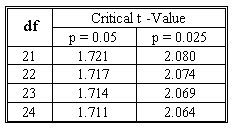### CFA Practice Question

There are 208 practice questions for this study session.

### CFA Practice Question

Beth has two independent variables and 25 observations in her regression analysis. The t-statistic for one of the independent variables X1 is 1.83. She wants to test H0: β1 >= 0 against H1: β1 < 0. What is the critical value for Student's t distribution and is the variable significant at the 5% confidence level?A. The critical value is 1.714, and the variable is significant.
B. The critical value is 1.717, and the variable is significant.
C. The critical value is -1.717 and the variable is not significant.
Explanation: Note that H1 is β1 < 0!
The degrees of freedom: 25 - 2 - 1 = 22.
Critical value -t0.05, 22: -1.717.
As -1.717 < 1.83, the variable is NOT significant at the 5% confidence level.